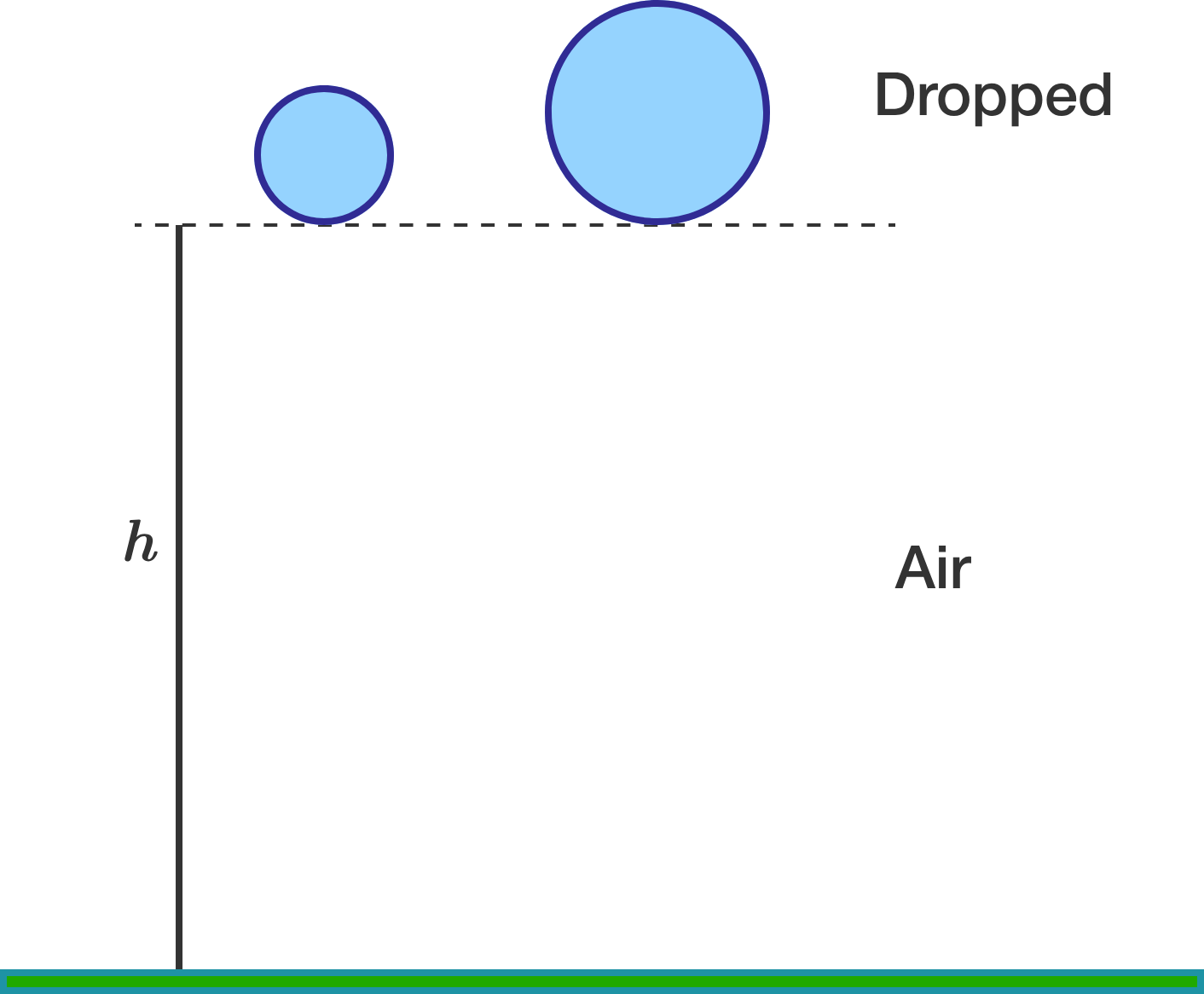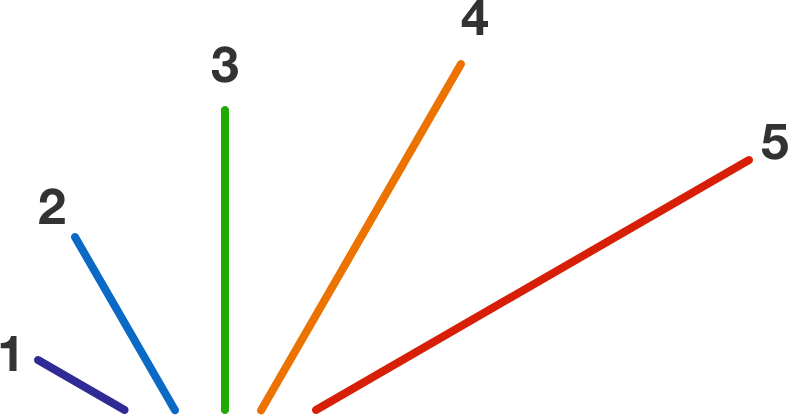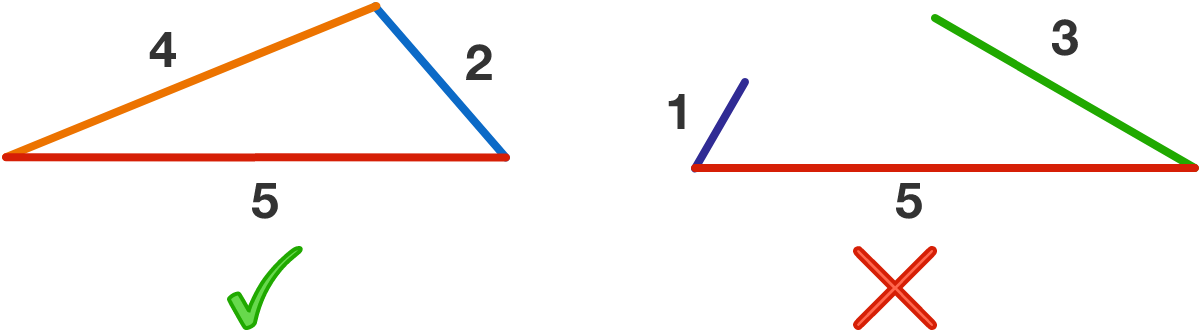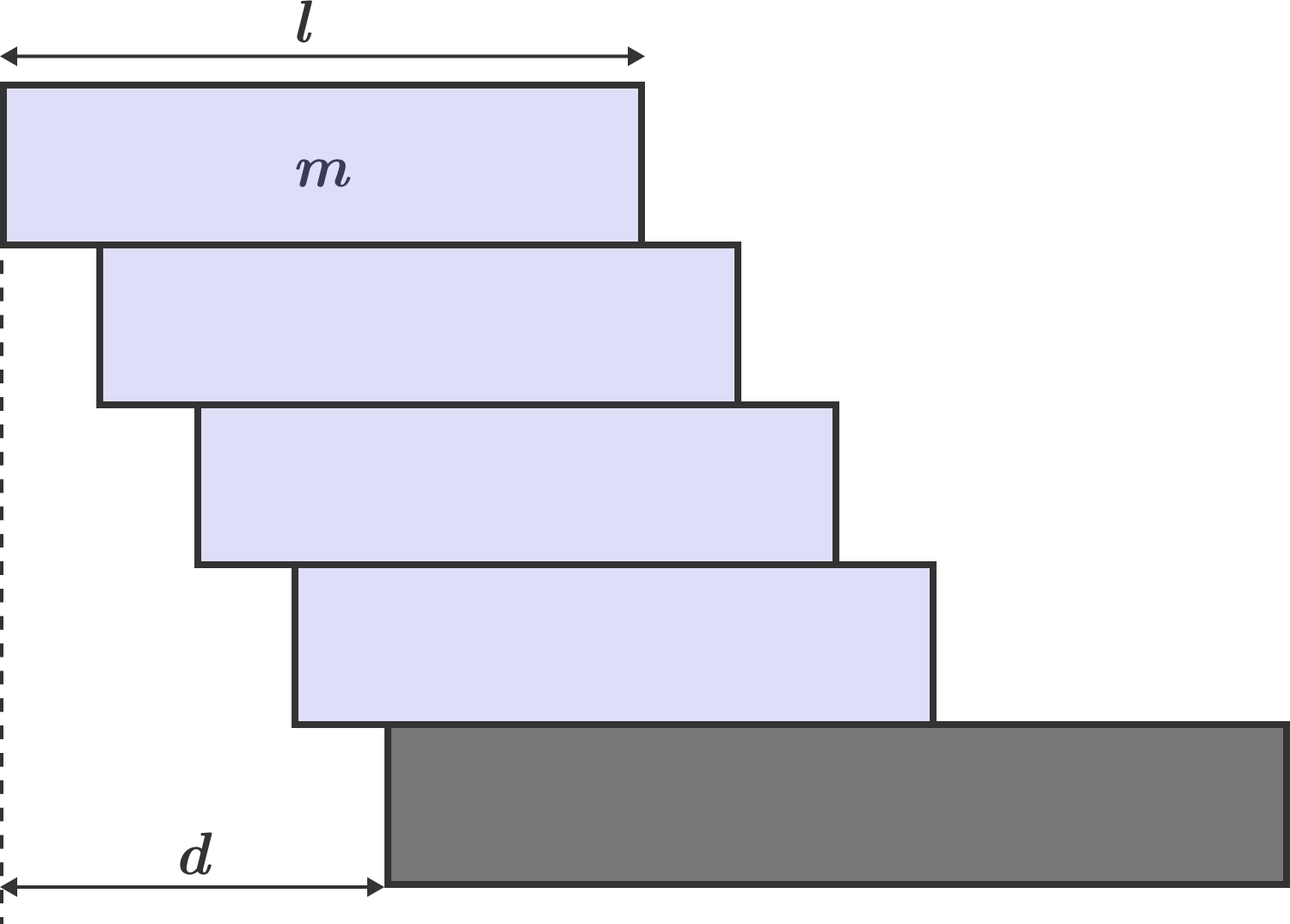Problems of the Week

Contribute a problem

2017-10-09 Intermediate

Sam drops two solid spheres (made of aluminum 6061 T6) with different radii simultaneously from the top of a tall building. Before the drop, the bottommost points of the spheres are aligned at the same height from the ground. The spheres fall through the air and hit the ground.

Which sphere will hit the ground first?A boat of length $2.5\text{ m}$ and weight $100\text{ kg}$ is standing still in the water. Tim weighs $70\text{ kg}$ and is inside the boat. If he walks from the stern to the prow of the boat, then by how much distance (in meters) will the boat move?


Details and Assumptions:

• Water resistance and tilt of the boat are negligible.
• Give your answer to 3 decimal places.

Sue and Kelly are playing a game with 5 sticks of lengths 1, 2, 3, 4, and 5. In this game, Sue randomly picks 2 of the sticks and Kelly deliberately picks 1 stick. In addition, Kelly can choose when she makes her pick (picking first, picking between Sue's picks, or picking last). Kelly's goal is to form a non-degenerate triangle from the 3 sticks chosen.Which sequence of picks will give Kelly the greatest chance of making a triangle?Roll a fair six-sided die until you roll a number that is less than one of your previous rolls. To three decimal places, what is the expected value of the number of rolls made?

Bonus: Suppose you rolled a fair $n$-sided die instead. What is the expected value of the number of rolls made?

Extra Bonus: As $n \to \infty,$ what does the expected value of the number of rolls approach?

On the edge of a wall, you build a brick tower that only holds because of the bricks' own weight. Your goal is to build a stable tower whose overhang $d$ is greater than the length $l$ of a single brick. What is the minimum number of bricks you need?

Note: Each brick has the same mass $m$ and uniform density. There is only one brick per layer. No gluing is allowed.Bonus Question: If enough bricks are available, can any desired value of the overhang be achieved? If so, how can the number of bricks required be estimated?

×